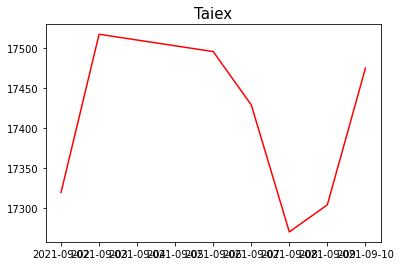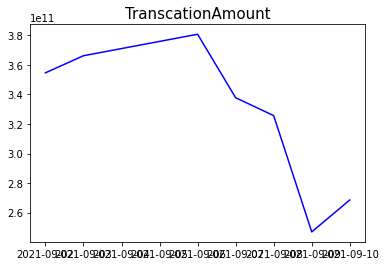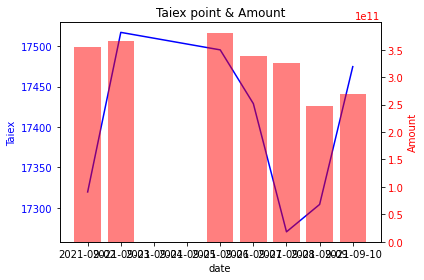DAY 12
0
Software Development

作法

首先：取得資料庫資料

# 取得資料庫的資料
conn.close() # 關閉連線

各自繪圖

# 畫圖
plt.plot(df["TradeDate"], df["Taiex"],"r")  # (x軸資料, y軸資料, 線的顏色)
plt.title("Taiex", {"fontsize":15})     # 設定圖的標題及文字大小# 畫圖
plt.plot(df["TradeDate"], df["TranscationAmount"],"b")  # (x軸資料, y軸資料, 線的顏色)
plt.title("TranscationAmount", {"fontsize":15})     # 設定圖的標題及文字大小合併圖表

fig, ax1 = plt.subplots()
plt.title("Taiex point & Amount")
plt.xlabel("date")
ax2 = ax1.twinx()

ax1.set_ylabel("Taiex", color="blue")
ax1.tick_params(axis="y", labelcolor="blue")

ax2.set_ylabel("Amount", color="r")
ax2.bar(df["TradeDate"], df["TranscationAmount"], color="r", alpha=0.5) # alpha: 增加透明度
ax2.tick_params(axis="y", labelcolor="r")

fig.tight_layout()
plt.show()